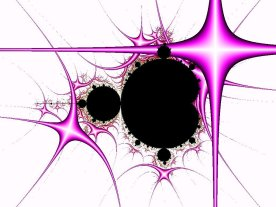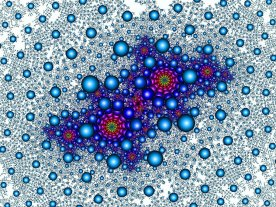VI. Orbit Traps This is one of the largest families of coloring algorithms, because it provides so many options for experimentation. In fact, entire software packages have been built specifically to explore this family of coloring algorithms. The basic idea is to choose a region of the complex plane (denoted by T) and watch the relationship between the zn values and T. T is usually defined as a central shape (usually a simply-calculated item, such as a point, line, or circle) and a threshold distance. Everything within the threshold distance of the trap is considered "inside" the trap. The earliest implementations of orbit trap algorithms simply watched for any zn that fell inside the trap, and at the first such value, stopped iterating and colored based on the distance to the central trap shape. (This is where the term "trap" comes from; once the orbit fell into T it was "trapped" and iteration stopped.)Figs 5a and 5b. Hypercross orbit trap in the Mandelbrot set (left). Gaussian integers algorithm in a Julia set (right). There are many more variations, however. The first class of variations covers the shape of the trap region, T. With the basic line and circle trap shapes, points of equal distance from the trap are the same shape as the trap, e.g. points equidistant from a line form a line. By using non-Euclidean distance measures, contours of equal distance can be transformed into other shapes; points (which with Euclidean distances would generate circular contours) can generate squares, astroids, and hyperbolas. Other interesting trap shapes can be created by using complex curves as the central trap shape, such as spirals and flowers. Furthermore, these trap shapes can be distorted by rotation, skewing, and stretching, often incrementally with each iteration. Another class of variations deals with the relationship between the distances of each zn to T. The classical implementation mentioned above stopped at the first zn within the threshold distance to T. Other variations use the last zn to enter the trap, or the closest, or the farthest that is still inside the trap. More exotic variations use different methods for combining all distances below the threshold together. The last major class of variations deals with the actual value used to produce the color. The most common method is simply the distance to the trap shape T, be it closest, farthest, first, etc. Other choices include the magnitude or angle of zn, or some combination of zn values related to the trap distances. With so many variations possible, and so many combinations of variations, it is nearly impossible to predict exactly what results will be achieved. Sometimes it is even difficult to tell a particular image has used the orbit trap algorithm at all. This is one reason this family of algorithms is so vastly popular. NEXT VisMath Home